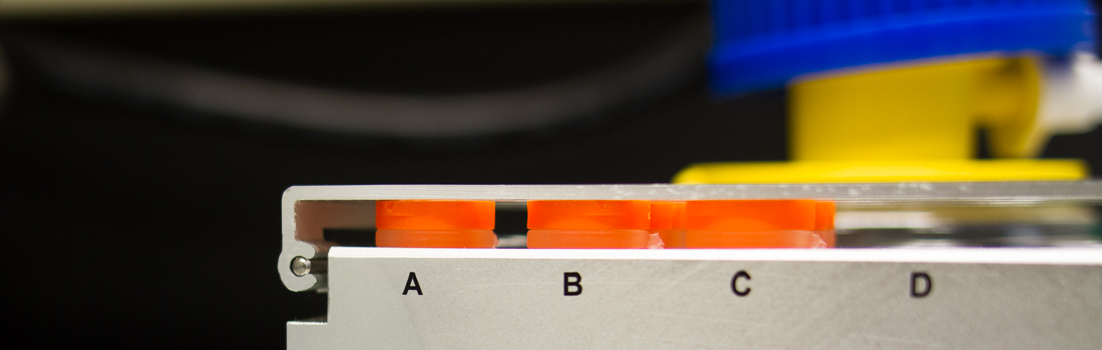# Biosensor Core# Thermodynamics of Binding

The energy used or expended in an equilibrium binding reaction is described by the equation,

ΔGo = ΔHo –TΔSo

where Go is the Gibbs Free Energy, Ho is enthalpy, T is the absolute temperature (in oK), and So is entropy.

The change in Gibbs Free Energy, ΔGo, is related to the dissociation constant for binding, KD, by the equation,

ΔGo = RT ln KD,

where R is the gas constant [1.98722 cal/oK•mol], and T is the absolute temperature.

Biacore® instrument measurements of KD as a function of temperature, T, can therefore reveal the enthalpy and entropy of any binding reaction, from the following relationship:

ln KD = ΔHo/RT – ΔSo/R

Thus, measuring KD as a function of T yields information about the thermodynamics of the binding reaction.